SYLLABUS  Previous: 6.2 Cubic-Interpolated Propagation (CIP)  Up: 6 LAGRANGIAN METHOD  Next: 6.4 Quiz

## 6.3 Non-Linear equations with CIP

Slide : [ CFL limit - Diffusion - Lagrangian - Non-linear || VIDEO login]

The same approach is applicable more generally for non-linear and vector equations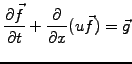(1)

where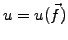and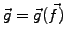. The problem is again decomposed in alternating phases with / without advection describing the evolution of the function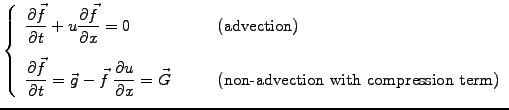(2)

and by differentiation of (eq.6.3#eq.1), the evolution of the derivative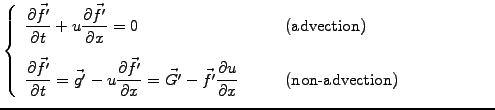(3)

Starting with the non-advection phase, the discretized function is first evolved according to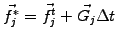(4)

where the super-script (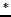) refers to an intermediate step. To avoid an explicit evaluation of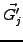, the equation for the derivative is computed with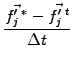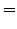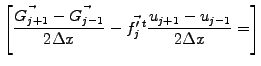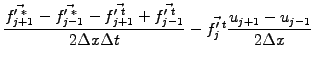(5)

The advection phase can then be evolved in the same manner as before (eq.6.2#eq.4), by shifting the cubic-Hermite polynomials along the characteristics (exercise 6.04).

SYLLABUS  Previous: 6.2 Cubic-Interpolated Propagation (CIP)  Up: 6 LAGRANGIAN METHOD  Next: 6.4 Quiz